# Special relativity, an introduction

Special relativity is a fundamental physics theory about space and time that was developed by Albert Einstein in 1905 as a modification of Newtonian physics. It was created to deal with some pressing theoretical and experimental issues in the physics of the time involving light and electrodynamics. The predictions of special relativity correspond closely to those of Newtonian physics at speeds which are low in comparison to that of light, but diverge rapidly for speeds which are a significant fraction of the speed of light. Special relativity has been experimentally tested on numerous occasions since its inception, and its predictions have been verified by those tests.

Einstein postulated that the speed of light is the same for all observers, irrespective of their motion relative to the light source. This was in total contradiction to classical mechanics, which had been accepted for centuries. Einstein's approach was based on thought experiments and calculations. In 1908, Hermann Minkowski reformulated the theory based on different postulates of a more geometrical nature. His approach depended on the existence of certain interrelations between space and time, which were considered completely separate in classical physics. This reformulation set the stage for further developments of physics.

Special relativity makes numerous predictions that are incompatible with Newtonian physics (and everyday intuition). The first such prediction described by Einstein is called the relativity of simultaneity, under which observers who are in motion with respect to each other may disagree on whether two events occurred at the same time or one occurred before the other. The other major predictions of special relativity are time dilation (under which a moving clock ticks more slowly than when it is at rest with respect the observer), length contraction (under which a moving rod may be found to be shorter than when it is at rest with respect to the observer), and the equivalence of mass and energy (written as E=mc2). Special relativity predicts a non-linear velocity addition formula, which prevents speeds greater than that of light from being observed. Special relativity also explains why Maxwell's equations of electromagnetism are correct in any frame of reference, and how an electric field and a magnetic field are two aspects of the same thing.

Special relativity has received experimental support in many ways, and it has been proven far more accurate than Newtonian mechanics. The most famous experimental support is the Michelson-Morley experiment, the results of which (showing that the speed of light is a constant) was one factor that motivated the formulation of the theory of special relativity. Other significant tests are the Fizeau experiment (which was first done decades before special relativity was proposed), the detection of the transverse Doppler effect, and the Haefele-Keating experiment. Today, scientists are so comfortable with the idea that the speed of light is always the same that the meter is now defined as being the distance traveled by light in 1/299,792,458th of a second. This means that the speed of light is now defined as being 299,792,458 m/s.

## Reference frames and Galilean relativity: A classical prelude

A reference frame is simply a selection of what constitutes stationary objects. Once the velocity of a certain object is arbitrarily defined to be zero, the velocity of everything else in the universe can be measured relative to it. When a train is moving at a constant velocity past a platform, one may either say that the platform is at rest and the train is moving or that the train is at rest and the platform is moving past it. These two descriptions correspond to two different reference frames. They are respectively called the rest frame of the platform and the rest frame of the train (sometimes simply the platform frame and the train frame).

The question naturally arises, can different reference frames be physically differentiated? In other words, can one conduct some experiments to claim that "we are now in an absolutely stationary reference frame?" Aristotle thought that all objects tend to cease moving and become at rest if there were no forces acting on them. Galileo challenged this idea and argued that the concept of absolute motion was unreal. All motion was relative. An observer who couldn't refer to some isolated object (if, say, he was imprisoned inside a closed spaceship) could never distinguish whether according to some external observer he was at rest or moving with constant velocity. Any experiment he could conduct would give the same result in both cases. However, accelerated reference frames are experimentally distinguishable. For example, if an astronaut moving in free space saw that the tea in his tea-cup was slanted rather than horizontal, he would be able to infer that his spaceship was accelerated. Thus not all reference frames are equivalent, but people have a class of reference frames, all moving at uniform velocity with respect to each other, in all of which Newton's first law holds. These are called the inertial reference frames and are fundamental to both classical mechanics and SR. Galilean relativity thus states that the laws of physics can not depend on absolute velocity, they must stay the same in any inertial reference frame. Galilean relativity is thus a fundamental principle in classical physics.

Mathematically, it says that if one transforms all velocities to a different reference frame, the laws of physics must be unchanged. What is this transformation that must be applied to the velocities? Galileo gave the common-sense "formula" for adding velocities: If

1. Particle P is moving at velocity v with respect to reference frame A and
2. Reference frame A is moving at velocity u with respect to reference frame B, then
3. The velocity of P with respect to B is given by v + u.

The formula for transforming coordinates between different reference frames is called the Galilean transformation. The principle of Galilean relativity then demands that laws of physics be unchanged if the Galilean transformation is applied to them. Laws of classical mechanics, like Newton's second law, obey this principle because they have the same form after applying the transformation. As Newton's law involves the derivative of velocity, any constant velocity added in a Galilean transformation to a different reference frame contributes nothing (the derivative of a constant is zero). Addition of a time-varying velocity (corresponding to an accelerated reference frame) will however change the formula (see pseudo force), since Galilean relativity only applies to non-accelerated inertial reference frames.

Time is the same in all reference frames because it is absolute in classical mechanics. All observers measure exactly the same intervals of time and there is such a thing as an absolutely correct clock.

## Invariance of length: The Euclidean picture

In special relativity, space and time are joined into a unified four-dimensional continuum called spacetime. To gain a sense of what spacetime is like, we must first look at the Euclidean space of Newtonian physics.

This approach to the theory of special relativity begins with the concept of "length." In everyday experience, it seems that the length of objects remains the same no matter how they are rotated or moved from place to place; as a result the simple length of an object doesn't appear to change or is "invariant." However, as is shown in the illustrations below, what is actually being suggested is that length seems to be invariant in a three-dimensional coordinate system.

The length of a line in a two-dimensional Cartesian coordinate system is given by Pythagoras' theorem: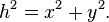$h^2 = x^2 + y^2. \,$

One of the basic theorems of vector algebra is that the length of a vector does not change when it is rotated. However, a closer inspection tells us that this is only true if we consider rotations confined to the plane. If we introduce rotation in the third dimension, then we can tilt the line out of the plane. In this case the projection of the line on the plane will get shorter. Does this mean length is not invariant? Obviously not. The world is three-dimensional and in a 3D Cartesian coordinate system the length is given by the three-dimensional version of Pythagoras's theorem:$k^2 = x^2 + y^2 + z^2. \,$Invariance in a 3D coordinate system: Pythagoras theorem gives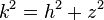$k^2 = h^2 + z^2$ but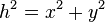$h^2 = x^2 + y^2$ therefore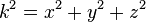$k^2 = x^2 + y^2 + z^2$. The length of an object is constant whether it is rotated or moved from one place to another in a 3D coordinate system

This is invariant under all rotations. The apparent violation of invariance of length only happened because we were "missing" a dimension. It seems that, provided all the directions in which an object can be tilted or arranged are represented within a coordinate system, the length of an object does not change under rotations. A 3-dimensional coordinate system is enough in classical mechanics because time is assumed absolute and independent of space in that context. It can be considered separately.

Note that invariance of length is not ordinarily considered a dynamic principle, not even a theorem. It is simply a statement about the fundamental nature of space itself. Space as we ordinarily conceive it is called a three-dimensional Euclidean space, because its geometrical structure is described by the principles of Euclidean geometry. The formula for distance between two points is a fundamental property of an Euclidean space, it is called the Euclidean metric tensor (or simply the Euclidean metric). In general, distance formulas are called metric tensors.

Note that rotations are fundamentally related to the concept of length. In fact, one may define length or distance to be that which stays the same (is invariant) under rotations, or define rotations to be that which keep the length invariant. Given any one, it is possible to find the other. If we know the distance formula, we can find out the formula for transforming coordinates in a rotation. If, on the other hand, we have the formula for rotations then we can find out the distance formula.

## The postulates of Special Relativity

Einstein developed Special Relativity on the basis of two postulates:

• First postulate—Special principle of relativity—The laws of physics are the same in all inertial frames of reference. In other words, there are no privileged inertial frames of reference.
• Second postulate—Invariance of c—The speed of light in a vacuum is independent of the motion of the light source.

Special Relativity can be derived from these postulates, as was done by Einstein in 1905. Einstein's postulates are still applicable in the modern theory but the origin of the postulates is more explicit. It was shown above how the existence of a universally constant velocity (the speed of light) is a consequence of modeling the universe as a particular four dimensional space having certain specific properties. The principle of relativity is a result of Minkowski structure being preserved under Lorentz transformations, which are postulated to be the physical transformations of inertial reference frames.

## The Minkowski formulation: Introduction of spacetime

Main article: Spacetime

After Einstein derived special relativity formally from the counterintuitive proposition that the speed of light is the same to all observers, the need was felt for a more satisfactory formulation. Minkowski, building on mathematical approaches used in non-Euclidean geometry and the mathematical work of Lorentz and Poincaré, realized that a geometric approach was the key. Minkowski showed in 1908 that Einstein's new theory could be explained in a natural way if the concept of separate space and time is replaced with one four-dimensional continuum called spacetime. This was a groundbreaking concept, and Roger Penrose has said that relativity was not truly complete until Minkowski reformulated Einstein's work.

The concept of a four-dimensional space is hard to visualize. It may help at the beginning to think simply in terms of coordinates. In three-dimensional space, one needs three real numbers to refer to a point. In the Minkowski space, one needs four real numbers (three space coordinates and one time coordinate) to refer to a point at a particular instant of time. This point at a particular instant of time, specified by the four coordinates, is called an event. The distance between two different events is called the spacetime interval.

A path through the four-dimensional spacetime, usually called Minkowski space, is called a world line. Since it specifies both position and time, a particle having a known world line has a completely determined trajectory and velocity. This is just like graphing the displacement of a particle moving in a straight line against the time elapsed. The curve contains the complete motional information of the particle.

In the same way as the measurement of distance in 3D space needed all three coordinates we must include time as well as the three space coordinates when calculating the distance in Minkowski space (henceforth called M). In a sense, the spacetime interval provides a combined estimate of how far two events occur in space as well as the time that elapses between their occurrence.

But there is a problem. Time is related to the space coordinates, but they are not equivalent. Pythagoras's theorem treats all coordinates on an equal footing (see Euclidean space for more details). We can exchange two space coordinates without changing the length, but we can not simply exchange a space coordinate with time, they are fundamentally different. It is an entirely different thing for two events to be separated in space and to be separated in time. Minkowski proposed that the formula for distance needed a change. He found that the correct formula was actually quite simple, differing only by a sign from Pythagoras's theorem: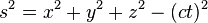$s^2 = x^2 + y^2 + z^2 - (ct)^2 \,$

where c is a constant and t is the time coordinate. Multiplication by c, which has the dimension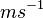$ms^{-1}$, converts the time to units of length and this constant has the same value as the speed of light. So the spacetime interval between two distinct events is given by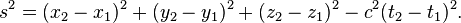$s^2 = (x_2 - x_1)^2 + (y_2 - y_1)^2 + (z_2 - z_1)^2 - c^2 (t_2 - t_1)^2. \,$

There are two major points to be noted. Firstly, time is being measured in the same units as length by multiplying it by a constant conversion factor. Secondly, and more importantly, the time-coordinate has a different sign than the space coordinates. This means that in the four-dimensional spacetime, one coordinate is different from the others and influences the distance differently. This new 'distance' may be zero or even negative. This new distance formula, called the metric of the spacetime, is at the heart of relativity. This distance formula is called the metric tensor of M. This minus sign means that a lot of our intuition about distances can not be directly carried over into spacetime intervals. For example, the spacetime interval between two events separated both in time and space may be zero (see below). From now on, the terms distance formula and metric tensor will be used interchangeably, as will be the terms Minkowski metric and spacetime interval.

In Minkowski spacetime the spacetime interval is the invariant length, the ordinary 3D length is not required to be invariant. The spacetime interval must stay the same under rotations, but ordinary lengths can change. Just like before, we were missing a dimension. Note that everything this far are merely definitions. We define a four-dimensional mathematical construct which has a special formula for distance, where distance means that which stays the same under rotations (alternatively, one may define a rotation to be that which keeps the distance unchanged).

Now comes the physical part. Rotations in Minkowski space have a different interpretation than ordinary rotations. These rotations correspond to transformations of reference frames. Passing from one reference frame to another corresponds to rotating the Minkowski space. An intuitive justification for this is given below, but mathematically this is a dynamical postulate just like assuming that physical laws must stay the same under Galilean transformations (which seems so intuitive that we don't usually recognize it to be a postulate).

Since by definition rotations must keep the distance same, passing to a different reference frame must keep the spacetime interval between two events unchanged. This requirement can be used to derive an explicit mathematical form for the transformation that must be applied to the laws of physics (compare with the application of Galilean transformations to classical laws) when shifting reference frames. These transformations are called the Lorentz transformations. Just like the Galilean transformations are the mathematical statement of the principle of Galilean relativity in classical mechanics, the Lorentz transformations are the mathematical form of Einstein's principle of relativity. Laws of physics must stay the same under Lorentz transformations. Maxwell's equations and Dirac's equation satisfy this property, and hence, they are relativistically correct laws (but classically incorrect, since they don't transform correctly under Galilean transformations).

With the statement of the Minkowski metric, the common name for the distance formula given above, the theoretical foundation of special relativity is complete. The entire basis for special relativity can be summed up by the geometric statement "changes of reference frame correspond to rotations in the 4D Minkowski spacetime, which is defined to have the distance formula given above." The unique dynamical predictions of SR stem from this geometrical property of spacetime. Special relativity may be said to be the physics of Minkowski spacetime. In this case of spacetime, there are six independent rotations to be considered. Three of them are the standard rotations on a plane in two directions of space. The other three are rotations in a plane of both space and time: These rotations correspond to a change of velocity, and are described by the traditional Lorentz transformations.

As has been mentioned before, one can replace distance formulas with rotation formulas. Instead of starting with the invariance of the Minkowski metric as the fundamental property of spacetime, one may state (as was done in classical physics with Galilean relativity) the mathematical form of the Lorentz transformations and require that physical laws be invariant under these transformations. This makes no reference to the geometry of spacetime, but will produce the same result. This was in fact the traditional approach to SR, used originally by Einstein himself. However, this approach is often considered to offer less insight and be more cumbersome than the more natural Minkowski formalism.

## Reference frames and Lorentz transformations: Relativity revisited

We have already discussed that in classical mechanics coordinate frame changes correspond to Galilean transfomations of the coordinates. Is this adequate in the relativistic Minkowski picture?

Suppose there are two people, Bill and John, on separate planets that are moving away from each other. Bill and John are on separate planets so they both think that they are stationary. John draws a graph of Bill's motion through space and time and this is shown in the illustration below:

John sees that Bill is moving through space as well as time but Bill thinks he is moving through time alone. Bill would draw the same conclusion about John's motion. In fact, these two views, which would be classically considered a difference in reference frames, are related simply by a coordinate transformation in M. Bill's view of his own world line and John's view of Bill's world line are related to each other simply by a rotation of coordinates. One can be transformed into the other by a rotation of the time axis. Minkowski geometry handles transformations of reference frames in a very natural way.

Changes in reference frame, represented by velocity transformations in classical mechanics, are represented by rotations in Minkowski space. These rotations are called Lorentz transformations. They are different from the Galilean transformations because of the unique form of the Minkowski metric. The Lorentz transformations are the relativistic equivalent of Galilean transformations. Laws of physics, in order to be relativistically correct, must stay the same under Lorentz transformations. The physical statement that they must be same in all inertial reference frames remains unchanged, but the mathematical transformation between different reference frames changes. Newton's laws of motion are invariant under Galilean rather than Lorentz transformations, so they are immediately recognizable as non-relativistic laws and must be discarded in relativistic physics. Schrödinger's equation is also non-relativistic.

Maxwell's equations are trickier. They are written using vectors and at first glance appear to transform correctly under Galilean transformations. But on closer inspection, several questions are apparent that can not be satisfactorily resolved within classical mechanics (see History of special relativity). They are indeed invariant under Lorentz transformations and are relativistic, even though they were formulated before the discovery of special relativity. Classical electrodynamics can be said to be the first relativistic theory in physics. To make the relativistic character of equations apparent, they are written using 4-component vector like quantities called 4-vectors. 4-Vectors transform correctly under Lorentz transformations. Equations written using 4-vectors are automatically relativistic. This is called the manifestly covariant form of equations. 4-Vectors form a very important part of the formalism of special relativity.

## Einstein's postulate: The constancy of the speed of light

Einstein's postulate that the speed of light is a constant comes out as a natural consequence of the Minkowski formulation

Proposition 1:

When an object is traveling at c in a certain reference frame, the spacetime interval is zero.

Proof:

The spacetime interval between the origin-event (0,0,0,0) and an event (x, y,z, t) is$s^2 = x^2 + y^2 + z^2 - (ct)^2 .\,$
The distance travelled by an object moving at velocity v for t seconds is: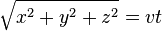$\sqrt{x^2 + y^2 + z^2} = vt \,$
giving$s^2 = (vt)^2 - (ct)^2 .\,$
Since the velocity v equals c we have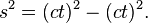$s^2 = (ct)^2 - (ct)^2 .\,$
Hence the spacetime interval between the events of departure and arrival is given by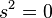$s^2 = 0 \,$

Proposition 2:

An object traveling at c in one reference frame is traveling at c in all reference frames.

Proof:

Let the object move with velocity v when observed from a different reference frame. A change in reference frame corresponds to a rotation in M. Since the spacetime interval must be conserved under rotation, the spacetime interval must be the same in all reference frames. In proposition 1 we showed it to be zero in one reference frame, hence it must be zero in all other reference frames. We get that$(vt)^2 - (ct)^2 = 0 \,$
which implies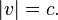$|v| = c .\,$

The paths of light rays have a zero spacetime interval, and hence all observers will obtain the same value for the speed of light. Therefore, when assuming that the universe has four dimensions that are related by Minkowski's formula, the speed of light appears as a constant, and does not need to be assumed (postulated) to be constant as in Einstein's original approach to special relativity.

## Clock delays and rod contractions: More on Lorentz transformations

Another consequence of the invariance of the spacetime interval is that clocks will appear to go slower on objects that are moving relative to you. This is very similar to how the 2D projection of a line rotated into the third-dimension appears to get shorter. Length is not conserved simply because we are ignoring one of the dimensions. Let us return to the example of John and Bill.

John observes the length of Bill's spacetime interval as: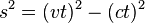$s^2 = (vt)^2 - (ct)^2 \,$

whereas Bill doesn't think he has traveled in space, so writes: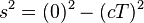$s^2 = (0)^2 - (cT)^2 \,$

The spacetime interval, s2, is invariant. It has the same value for all observers, no matter who measures it or how they are moving in a straight line. This means that Bill's spacetime interval equals John's observation of Bill's spacetime interval so: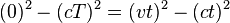$(0)^2 - (cT)^2 = (vt)^2 - (ct)^2 \,$

and$-(cT)^2 = (vt)^2 - (ct)^2 \,$

hence$t = \frac{T}{\sqrt{1 - \frac{v^2}{c^2}}} \,$.

So, if John sees a clock that is at rest in Bill's frame record one second, John will find that his own clock measures between these same ticks an interval t, called coordinate time, which is greater than one second. It is said that clocks in motion slow down, relative to those on observers at rest. This is known as "relativistic time dilation of a moving clock." The time that is measured in the rest frame of the clock (in Bill's frame) is called the proper time of the clock.

In special relativity, therefore, changes in reference frame affect time also. Time is no longer absolute. There is no universally correct clock, time runs at different rates for different observers.

Similarly it can be shown that John will also observe measuring rods at rest on Bill's planet to be shorter in the direction of motion than his own measuring rods. This is a prediction known as "relativistic length contraction of a moving rod." If the length of a rod at rest on Bill's planet is$X$, then we call this quantity the proper length of the rod. The length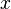$x$ of that same rod as measured on John's planet, is called coordinate length, and given by$x = X \sqrt{1 - \frac{v^2}{c^2}} \,$.

These two equations can be combined to obtain the general form of the Lorentz transformation in one spatial dimension: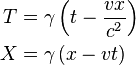\begin{align}T &= \gamma \left( t - \frac{v x}{c^{2}} \right) \\ X &= \gamma \left( x - v t \right)\\\end{align}

or equivalently: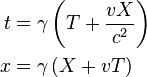\begin{align}t &= \gamma \left( T + \frac{v X}{c^{2}} \right) \\ x &= \gamma \left( X + v T \right)\\\end{align}

where the Lorentz factor is given by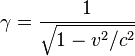$\gamma = { 1 \over \sqrt{1 - v^2/c^2} }$

The above formulas for clock delays and length contractions are special cases of the general transformation.

Alternatively, these equations for time dilation and length contraction (here obtained from the invariance of the spacetime interval), can be obtained directly from the Lorentz transformation by setting X = 0 for time dilation, meaning that the clock is at rest in Bill's frame, or by setting t = 0 for length contraction, meaning that John must measure the distances to the end points of the moving rod at the same time.

A consequence of the Lorentz transformations is the modified velocity-addition formula:$s = {v+u \over 1+(v/c)(u/c)}.$

## Simultaneity and clock desynchronization

Rather counter-intuitively, special relativity suggests that when 'at rest' we are actually moving through time at the speed of light. As we speed up in space we slow down in time. At the speed of light in space, time slows down to zero. This is a rotation of the time axis into the space axis. We observe that the object speeding by relativistically as having its time axis not at a right angle.

The consequence of this in Minkowski's spacetime is that clocks will appear to be out of phase with each other along the length of a moving object. This means that if one observer sets up a line of clocks that are all synchronized so they all read the same time, then another observer who is moving along the line at high speed will see the clocks all reading different times. This means that observers who are moving relative to each other see different events as simultaneous. This effect is known as "Relativistic Phase" or the "Relativity of Simultaneity." Relativistic phase is often overlooked by students of special relativity, but if it is understood, then phenomena such as the twin paradox are easier to understand.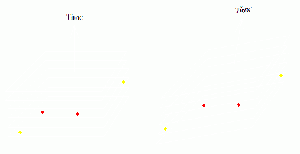The "plane of simultaneity" or "surface of simultaneity" contains all those events that happen at the same instant for a given observer. Events that are simultaneous for one observer are not simultaneous for another observer in relative motion.

Observers have a set of simultaneous events around them that they regard as composing the present instant. The relativity of simultaneity results in observers who are moving relative to each other having different sets of events in their present instant.

The net effect of the four-dimensional universe is that observers who are in motion relative to you seem to have time coordinates that lean over in the direction of motion, and consider things to be simultaneous that are not simultaneous for you. Spatial lengths in the direction of travel are shortened, because they tip upwards and downwards, relative to the time axis in the direction of travel, akin to a rotation out of three-dimensional space.

Great care is needed when interpreting spacetime diagrams. Diagrams present data in two dimensions, and cannot show faithfully how, for instance, a zero length spacetime interval appears.

## Mass Velocity Relationship

E = mc2 where m stands for rest mass (invariant mass), applies most simply to single particles with no net momentum. But it also applies to ordinary objects composed of many particles so long as the particles are moving in different directions so the total momentum is zero. The mass of the object includes contributions from heat and sound, chemical binding energies and trapped radiation. Familiar examples are a tank of gas, or a hot bowl of soup. The kinetic energy of their particles, the heat motion and radiation, contribute to their weight on a scale according to E = mc2.

The formula is the special case of the relativistic energy-momentum relationship: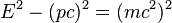$\, E^2 - (pc)^2 = (m c^2)^2$

This equation gives the rest mass of a system which has an arbitrary amount of momentum and energy. The interpretation of this equation is that the rest mass is the relativistic length of the energy-momentum four-vector.

If the equation$E=mc^2$ is used with the rest mass of the object, the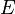$E$ given by the equation will be the rest energy of the object, and will change with according to the object's internal energy, heat and sound and chemical binding energies, but will not change with the object's overall motion).

If the equation$E=mc^2$ is used with the relativistic mass of the object, the energy will be the total energy of the object, which is conserved in collisions with other fast moving objects.

In developing special relativity, Einstein found that the total energy of a moving body is$E = \frac{m_0 c^2}\sqrt{1-\frac{v^2}{c^2}},$

with$v$ the velocity.

For small velocities, this reduces to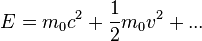$E = m_0 c^2 + \frac{1}{2}m_0 v^2 + ...$

Which includes the newtonian kinetic energy, as expected, but also an enormous constant term, which is not zero when the object isn't moving.

The total momentum is: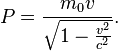$P = \frac{m_0 v}\sqrt{1-\frac{v^2}{c^2}}.$

The ratio of the momentum to the velocity is the relativistic mass, and this ratio is equal to the total energy times c2. The energy and relativistic mass are always related by the famous formula.

While this is suggestive, it does not immediately imply that the energy and mass are equivalent because the energy can always be redefined by adding or subtracting a constant. So it is possible to subtract the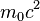$m_0 c^2$ from the expression for$E$ and this is also a valid conserved quantity, although an ugly one. Einstein needed to know whether the rest-mass of the object is really an energy, or whether the constant term was just a mathematical convenience with no physical meaning.

In order to see if the$m_0 c^2$ is physically significant, Einstein considered processes of emission and absorption. He needed to establish that an object loses mass when it emits energy. He did this by analyzing two photon emission in two different frames.

After Einstein first made his proposal, it became clear that the word mass can have two different meanings. The rest mass is what Einstein called m, but others defined the relativistic mass as: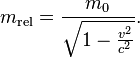$m_{\mathrm{rel}} = \frac{m_0}{\sqrt{1-\frac{v^2}{c^2}}} .$

This mass is the ratio of momentum to velocity, and it is also the relativistic energy divided by c2. So the equation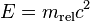$E=m_{\mathrm{rel}}c^2$ holds for moving objects. When the velocity is small, the relativistic mass and the rest mass are almost exactly the same.$E = mc^2$ either means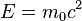$E = m_0 c^2$ for an object at rest, or$E = m_{\mathrm{rel}} c^2$ when the object is moving.

Einstein's original papers treated m as what would now be called the rest mass and some claim that he did not like the idea of "relativistic mass." When modern physicists say "mass," they are usually talking about rest mass, since if they meant "relativistic mass," they would just say "energy."

We can rewrite the expression for the energy as a Taylor series: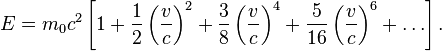$E = m_0 c^2 \left[1 + \frac{1}{2} \left(\frac{v}{c}\right)^2 + \frac{3}{8} \left(\frac{v}{c}\right)^4 + \frac{5}{16} \left(\frac{v}{c}\right)^6 + \ldots \right].$

For speeds much smaller than the speed of light, higher-order terms in this expression get smaller and smaller because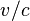$v/c$ is small. For low speeds we can ignore all but the first two terms: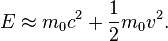$E \approx m_0 c^2 + \frac{1}{2} m_0 v^2 .$

The total energy is a sum of the rest energy and the Newtonian kinetic energy.

The classical energy equation ignores both the$m_0 c^2$ part, and the high-speed corrections. This is appropriate, because all the high order corrections are small. Since only changes in energy affect the behavior of objects, whether we include the$m_0 c^2$ part makes no difference, since it is constant. For the same reason, it is possible to subtract the rest energy from the total energy in relativity. In order to see if the rest energy has any physical meaning, it is essential to consider emission and absorption of energy in different frames.

The higher-order terms are extra correction to Newtonian mechanics which become important at higher speeds. The Newtonian equation is only a low speed approximation, but an extraordinarily good one. All of the calculations used in putting astronauts on the moon, for example, could have been done using Newton's equations without any of the higher order corrections.

## Mass-energy equivalence: Sunlight and atom bombs

Einstein showed that mass is simply another form of energy. The energy equivalent of rest mass m is E = mc2. This equivalence implies that mass should be interconvertible with other forms of energy. This is the basic principle behind atom bombs and production of energy in nuclear reactors and stars (like Sun).

The standard model of the structure of matter has it that most of the 'mass' of the atom is in the atomic nucleus, and that most of this nuclear mass is in the intense field of light-like gluons swathing the quarks. Most of what is called the mass of an object is thus already in the form of energy, the energy of the quantum color field that confines the quarks.

The sun, for instance, fuels its prodigious output of energy by converting each second 600 billion kilograms of hydrogen-1 (single proton]]s) into 595.2 billion kilograms of helium-4 (2 protons combined with 2 neutrons)—the 4.2 billion kilogram difference is the energy which the sun radiates into space each second. The sun, it is estimated, will continue to turn 4.2 billion kilos of mass into energy for the next 5 billion years or so before leaving the main sequence.

The atomic bombs that ended the Second World War, in comparison, converted about a thirtieth of an ounce of mass into energy.

The energy involved in chemical reactions is so small, however, that the conservation of mass is an excellent approximation.

## General relativity: A peek forward

Unlike Newton's laws of motion, relativity is not based upon dynamical postulates. It does not assume anything about motion or forces. Rather, it deals with the fundamental nature of spacetime. It is concerned with describing the geometry of the backdrop on which all dynamical phenomena take place. In a sense therefore, it is a meta-theory, a theory that lays out a structure that all other theories must follow. In truth, Special relativity is only a special case. It assumes that spacetime is flat. That is, it assumes that the structure of Minkowski space and the Minkowski metric tensor is constant throughout. In General relativity, Einstein showed that this is not true. The structure of spacetime is modified by the presence of matter. Specifically, the distance formula given above is no longer generally valid except in space free from mass. However, just like a curved surface can be considered flat in the infinitesimal limit of calculus, a curved spacetime can be considered flat at a small scale. This means that the Minkowski metric written in the differential form is generally valid.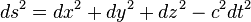$ds^2 = dx^2 + dy^2 + dz^2 - c^2 dt^2 \,$

One says that the Minkowski metric is valid locally, but it fails to give a measure of distance over extended distances. It is not valid globally. In fact, in general relativity the global metric itself becomes dependent on the mass distribution and varies through space. The central problem of general relativity is to solve the famous Einstein field equations for a given mass distribution and find the distance formula that applies in that particular case. Minkowski's spacetime formulation was the conceptual stepping stone to general relativity. His fundamentally new outlook allowed not only the development of general relativity, but also to some extent quantum field theories.